# Computing with Graphs

Graphs are quite ubiquitous in computing. Many everyday and practical problems can be modelled on graphs. We'll start out by looking at how to construct a few kinds of graphs and then discuss some fundamental algorithms we can use with them. To actually carry out the computations, we'll be using a Python module called NetworkX.

## Introduction and Some Model Problems

Recall that a graph consists of a collection of nodes and a collection of edges between them. Graphs typically come in two flavors: undirected and directed, each having their own purpose.

As a sort of look ahead, let's think of a few model problems where each type of graph may occur.

Our first problem deals with an internet service provider who would like to expand its services and provide fiber optic internet to a town. Of course, they want to do this at the lowest cost.

In order to figure out the best way to do this, we'll model the locations we must connect as nodes in a graph and potential lines of cable to run as (undirected) edges. In addition, these edges are weighted by a cost due to things such as the cost of cable or the labor that goes into digging trenches for the cable. How should we solve this problem?

Of course, this is a rather simplified version of a more realistic problem, as we're focused on a narrow sense of connectivity. A more reasonable requirement would build in some redundency. For example, if a cable were damaged, the entire network wouldn't go down.

Our second problem deals with scheduling. What we've got is a bunch a tasks which must be completed; the catch is that some of the tasks can't be started until other ones are complete. For example, if I'm building a house, I must excavate the ground before laying the foundation. On the other hand, determining the kind of lights or wallpaper I'd like is completely independent and someone else can do this simultaniously.

We'll model this problem as a directed graph where the tasks are the nodes and the directed edges encode dependencies between tasks. So, maybe I have an edge "Task A -> Task B" if "Task B" requires "Task A" to be completed first. We'll see that there is a nice algorithm called topological sorting which gives us an ordered list of tasks which ensures that all dependencies as we complete the list.

## Creating and Drawing Graphs

First, we'll look at the Graph and DiGraph in NetworkX to create some simple graphs. We'll start by importing NetworkX and building a simple cyclic graph on three nodes.

In :
%matplotlib inline
import networkx as nx

In :
G = nx.Graph()

plt.figure()
nx.draw(G, with_labels=True)
plt.show()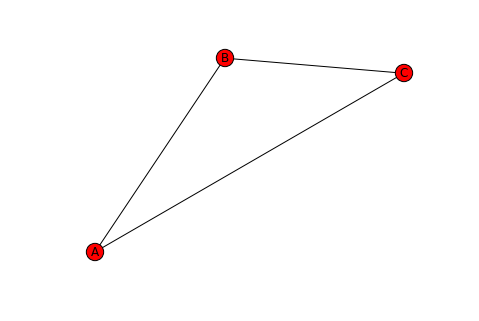Not bad! Unfortunately, that was a lot of typing for such a simple graph. Let's look at some functions to make this more succinct.

In :
G = nx.Graph()

G.add_edges_from([('A', 'B'), ('A', 'C'), ('B', 'C')])

plt.figure()
nx.draw(G, with_labels=True)
plt.show()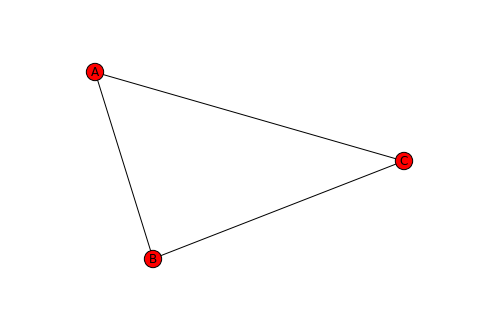Now, what if we want an undirected graph? We just change Graph to DiGraph.

In :
G = nx.DiGraph()

G.add_edges_from([('A', 'B'), ('B', 'C'), ('C', 'A')])

plt.figure()
nx.draw(G, with_labels=True)
plt.show()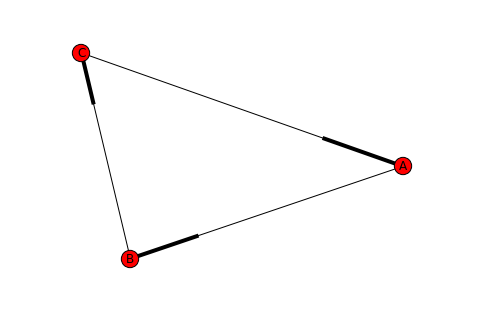Notice the difference in how the edges are rendered! Now the order matters!

In :
G = nx.DiGraph()

plt.figure()
nx.draw(G, with_labels=True)
plt.show()

G = nx.DiGraph()

plt.figure()
nx.draw(G, with_labels=True)
plt.show()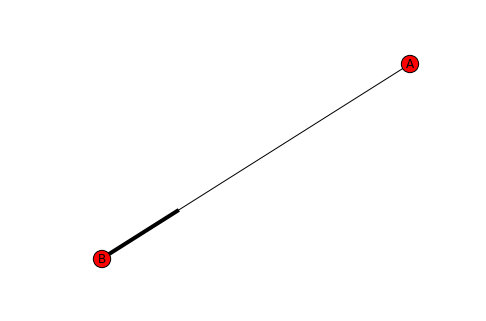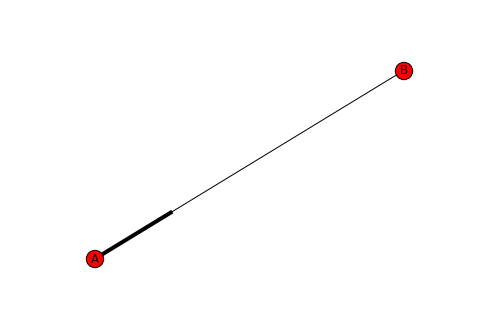## Exercise 1

1. Try creating a directed "circle" graph with $n$ nodes "by hand". The graph should have nodes $1, 2, \ldots, n$ with edges between $(1,2), (2, 3), \ldots, (n-1,n), (n,1)$.

2. Try creating a complete graph on 5 nodes. (A complete graph is an undirected graph with an edge between every pair of nodes.)

We'll see that there some helper functions to do this kind of thing in a moment, but consider this a warm-up.

## Aside: Common Construction

NetworkX provides a few constructors for commonly occuring graphs. For example:

In :
G = nx.complete_graph(6)

plt.figure()
nx.draw_circular(G)
plt.show()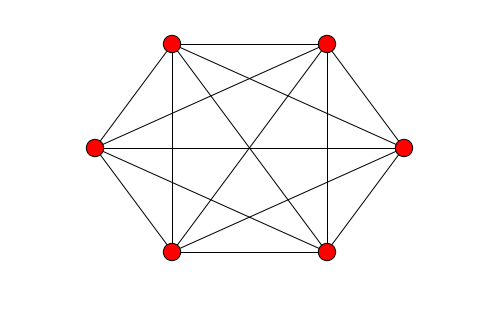In :
G = nx.cycle_graph(13)

plt.figure()
nx.draw_circular(G)
plt.show()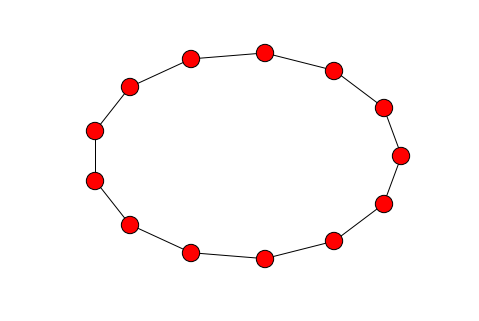There's even some rather exotic ones!

In :
G = nx.lollipop_graph(5, 2)

plt.figure()
nx.draw(G)
plt.show()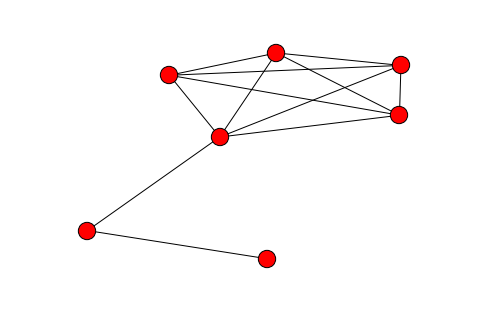## Fundamental Graph Algorithms

We'll discuss a few fundamental graph algorithms: graph traversal, shortest path, minimal spanning tree and max flow/min cut. There are many, many others, but we'll focus on these here.

## Graph Traversal

Graph traversal provides us with a way to explore a graph some some starting node. One example you can think about is, if we're stuck in a maze, graph traversal would allow us to explore until we found an exit. (But in a principled way...not just randomly wandering around.)

In some sense, we saw this much earlier as the challenge problem during the second day in our implementation of depth-first search. Let's see how we can leverage NetworkX to do this for us. We'll also see how to easily change to an alternative search strategy known as breadth-first search.

We'll start with a "double diamond" graph this time:

In :
G = nx.DiGraph()

G.add_nodes_from([0, 1, 2, 3, 4, 5, 6])
(0, 1),
(0, 2),
(1, 3),
(2, 3),
(3, 4),
(3, 5),
(4, 6),
(5, 6),
])

plt.figure()
nx.draw(G, with_labels=True)
plt.show()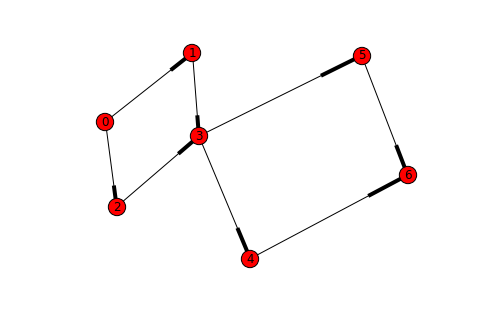Now we'll use the built-in NetworkX algorithms.

In :
T = nx.depth_first_search.dfs_tree(G, 0)

plt.figure()
nx.draw(T, with_labels=True)
plt.show()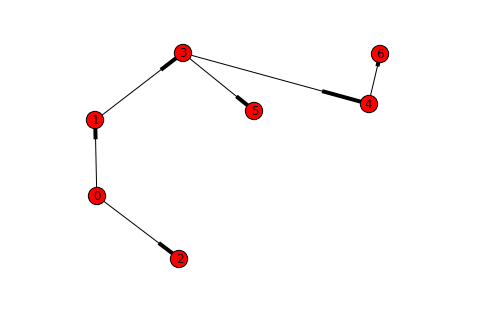In :
T = nx.depth_first_search.dfs_tree(G, 1)

plt.figure()
nx.draw(T, with_labels=True)
plt.show()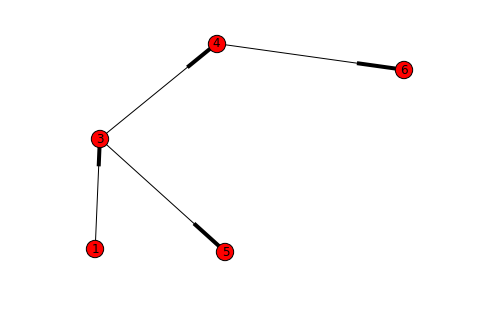In :
T = nx.depth_first_search.dfs_tree(G, 3)

plt.figure()
nx.draw(T, with_labels=True)
plt.show()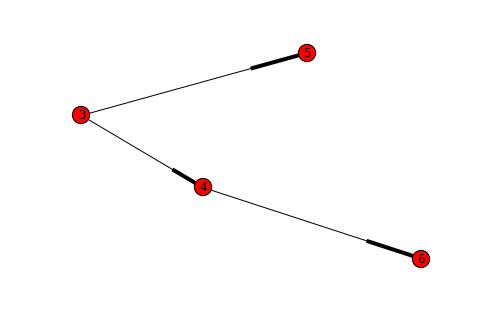By changing one line of code, we can also employ a different search method:

In :
T = nx.breadth_first_search.bfs_tree(G, 0)

plt.figure()
nx.draw(T, with_labels=True)
plt.show()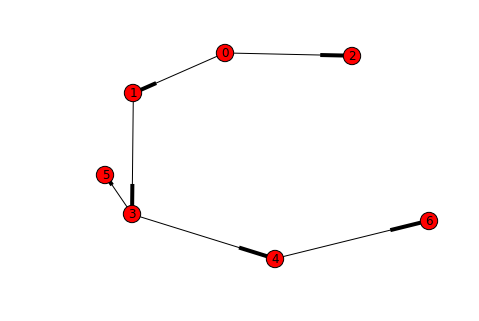## Shortest Path

This one speaks for itself. How many times have you wanted to know the shortest route to get from point A to point B?

We'll model this problem on either an undirected or directed graph with weighted edges.

Shortest path also tends to come in two flavors:

The first is single-source shortest path. This computes the shortest paths to all other nodes from a particular starting node. For example, compute the best route from a starting location to and ending location is typically done this way. The classic algorithm for this is Dijkstra's algorithm. This also tends to be a good problem to see why "greedy" algorithms don't work. That is, just repeatedly choosing the shortest edge you can see.

The second flavor is the all-pairs shortest path problem. This time, we want to compute the shortest path between any two pairs of nodes on the graph. This is typically done with the Floyd-Warshall algorithm.

With the background out of the way, let's see how we can represent a weighted graph and compute the shortest path. I'll be using the example from the Dijkstra's Wikipedia page.

In :
G = nx.Graph()

G.add_nodes_from([1, 2, 3, 4, 5, 6])
(1, 2, 7),
(2, 4, 15),
(4, 5, 6),
(5, 6, 9),
(6, 1, 14),
(1, 3, 9),
(2, 3, 10),
(3, 4, 11),
(3, 6, 2),
], weight='distance') # call the additional weight values 'distance'

Out:
{1: 0, 2: 7, 3: 9, 4: 20, 5: 20, 6: 11}

Now we've defined the graph. What I'd done in defining the edges is added a third argument which I'm calling the 'distance'. We'll see how that's used now:

In :
S = nx.shortest_path_length(G, 1, weight='distance') # use those 'distance' values here
S

Out:
{1: 0, 2: 7, 3: 9, 4: 20, 5: 20, 6: 11}

This computes the distance between my starting node 1 and all the other nodes in the graph. If wanted to actual paths rather than just the distance, I can use:

In :
P = nx.shortest_path(G, 2)
P

Out:
{1: [2, 1], 2: , 3: [2, 3], 4: [2, 4], 5: [2, 4, 5], 6: [2, 1, 6]}

So, we can see that the shortest path from 1 to 5 goes through 1 -> 2 -> 4 -> 5.

## Minimal Spanning Tree

One of our motivating examples regarded an internet service provider who was laying down fiber optic cable to expand its services. We'll look a litte more carefully at how they may solve this problem.

Let's construct a simple "crossed-box" graph with weighted edges and try to compute a spanning tree of minimum weight in order to connect the network.

First we'll suppose that the weights come purely from the geometry of the box.

In :
G = nx.Graph()

G.add_nodes_from([0, 1, 2, 3])
(0, 1, 1.0),
(1, 2, 1.0),
(2, 3, 1.0),
(3, 0, 1.0),
(0, 2, 2.0**.5),
(1, 3, 2.0**.5),
])

T = nx.minimum_spanning_tree(G)

plt.figure()
nx.draw(G, with_labels=True)
plt.show()

plt.figure()
nx.draw(T, with_labels=True)
plt.show()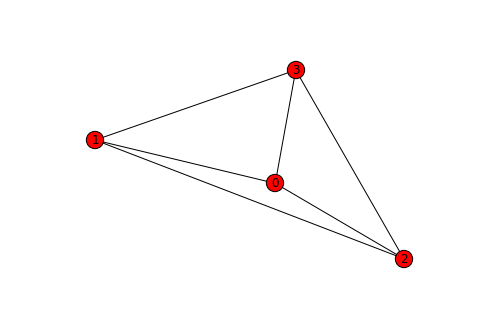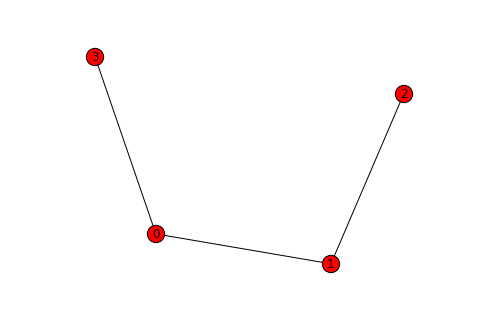Now, we suppose that there was already some infrastructure we could use to run cable from node 0 to 2. Let's see how that affects our solution.

In :
G.edge['weight'] = 0.4    # update the weight to take into account availible infrastructure

T = nx.minimum_spanning_tree(G) # recompute minimal spanning tree

plt.figure()
nx.draw(T, with_labels=True)
plt.show()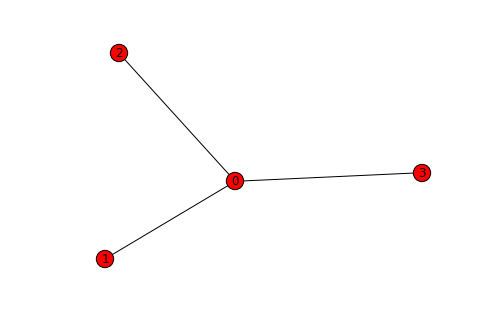## Max Flow / Min Cut

This time, we'll change terminology a little bit. We'll consider a graph which has certain capacities on its edges. For example, a road network may only be able to support a maximum amount of traffic. Or, a compute network may have limited bandwidth.

The goal is, we have two specials nodes called the source and the sink and we want to compute the maximum amount of "stuff" we can transport from the source to the sink. The constraint is, we cannot exceed the capacities of the edges and any node we use along the way must have the same amount of incoming "stuff" and outgoing "stuff".

If you'd like a more formal reference, take a look at this.

Let's try this on the simplest example: Just a single path!

In :
G = nx.DiGraph()

G.add_nodes_from([0, 1, 2, 3])

(0, 1, 4),
(1, 2, 3),
(2, 3, 6),
], weight='capacity')

plt.figure()
nx.draw(G, with_labels=True)
plt.show()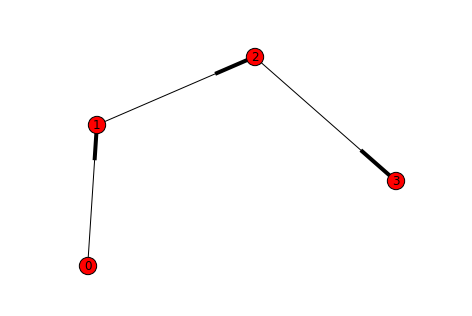On this graph, it's easy to see that the bottleneck is the edge 1 -> 2 which has capacity 3. Let's check this!

In :
nx.maximum_flow_value(G, 0, 3)

Out:
3

It turns out the this algorithm is quite broad in its application. I'll try to post some references to surprising applications of this. We'll see one easy example in today's projects.